# Sets, Relations and Binary Operations Notes Class 11th Maths

0
1569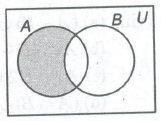## Set

Set is a collection of well-defined objects which are distinct from each other. Sets are usually denoted by capital letters A, B,C,… and elements are usually denoted by small letters a, b,c,… .

If ‘a’ is an element of a set A, then we write a ∈ A and say ‘a’ belongs to A or ‘a’ is in A or ‘a’ is a member of A. If ‘a’ does not belongs to A, we write a ∉ A.

Standard Notations

• N : A set of natural numbers.
• W : A set of whole numbers.
• Z : A set of integers.
• Z+/Z : A set of all positive/negative integers.
• Q : A set of all rational numbers.
• Q+/Q : A set of all positive/ negative rational numbers.
• R : A set of real numbers.
• R+/R: A set of all positive/negative real numbers.
• C : A set of all complex numbers.

Methods for Describing a Set

(i) Roster/Listing Method/Tabular Form In this method, a set is described by listing element, separated by commas, within braces.
e.g., A = {a, e, i, o, u}

(ii) Set Builder/Rule Method In this method, we write down a property or rule which gives us all the elements of the set by that rule.
e.g.,A = {x : x is a vowel of English alphabets}

Types of Sets

1. Finite Set A set containing finite number of elements or no element.
2. Cardinal Number of a Finite Set The number of elements in a given finite set is called cardinal number of finite set, denoted by n (A).
3. Infinite Set A set containing infinite number of elements.
4. Empty/Null/Void Set A set containing no element, it is denoted by (φ) or { }.
5. Singleton Set A set containing a single element.
6. Equal Sets Two sets A and B are said to be equal, if every element of A is a member of B and every element of B is a member of A and we write A = B.
7. Equivalent Sets Two sets are said to be equivalent, if they have same number of elements.
If n(A) = n(B), then A and B are equivalent sets. But converse is not true.
8. Subset and Superset Let A and B be two sets. If every element of A is an element of B, then A is called subset of B and B is called superset of A. Written as
A ⊆ B or B ⊇ A
9. Proper Subset If A is a subset of B and A ≠ B, then A is called proper subset of B and we write A ⊂ B.
10. Universal Set (U) A set consisting of all possible elements which occurs under consideration is called a universal set.
11. Comparable Sets Two sets A and Bare comparable, if A ⊆ B or B ⊆ A.
12. Non-Comparable Sets For two sets A and B, if neither A ⊆ B nor B ⊆ A, then A and B are called non-comparable sets.
13. Power Set (P) The set formed by all the subsets of a given set A, is called power set of A, denoted by P(A).
14. Disjoint Sets Two sets A and B are called disjoint, if, A ∩ B = (φ).

Venn Diagram

In a Venn diagram, the universal set is represented by a rectangular region and a set is represented by circle or a closed geometrical figure inside the universal set.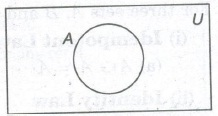Operations on Sets

1. Union of Sets

The union of two sets A and B, denoted by A ∪ B is the set of all those elements, each one of which is either in A or in B or both in A and B.2. Intersection of Sets

The intersection of two sets A and B, denoted by A ∩ B, is the set of all those elements which are common to both A and B.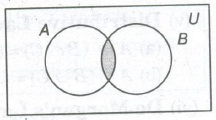If A1, A2,… , An is a finite family of sets, then their intersection is denoted by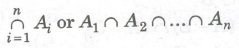3. Complement of a Set

If A is a set with U as universal set, then complement of a set, denoted by A’ or Ac is the set U – A .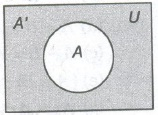4. Difference of Sets

For two sets A and B, the difference A – B is the set of all those elements of A which do not belong to B.5. Symmetric Difference

For two sets A and B, symmetric difference is the set (A – B) ∪ (B – A) denoted by A Δ B.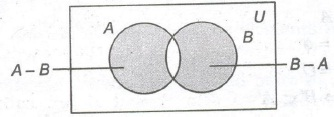Laws of Algebra of Sets

For three sets A, B and C

(i) Commutative Laws

A ∩ B = B ∩ A
A ∪ B = B ∪ A

(ii) Associative Laws

(A ∩ B) ∩ C = A ∩ (B ∩ C)
(A ∪ B) ∪ C = A ∪ (B ∪ C)

(iii) Distributive Laws

A ∩ (B ∪ C) = (A ∩ B) ∪ (A ∩ C)
A ∪ (B ∩ C) = (A ∪ B) ∩ (A ∪ C)

(iv) Idempotent Laws

A ∩ A = A
A ∪ A = A

(v) Identity Laws

A ∪ Φ = A
A ∩ U = A

(vi) De Morgan’s Laws

(a) (A ∩ B) ′ = A ′ ∪ B ′
(b) (A ∪ B) ′ = A ′ ∩ B ′
(c) A – (B ∩ C) = (A – B) ∩ (A- C)
(d) A – (B ∪ C) = (A – B) ∪ ( A – C)

(vii) (a) A – B = A ∩ B’
(b) B – A = B ∩ A’
(c) A – B = A ⇔A ∩ B= (Φ)
(d) (A – B) ∪ B= A ∪ B
(e) (A – B) ∩ B = (Φ)
(f) A ∩ B ⊆ A and A ∩ B ⊆ B
(g) A ∪ (A ∩ B)= A
(h) A ∩ (A ∪ B)= A

(viii) (a) (A – B) ∪ (B – A) = (A ∪ B) – (A ∩ B)
(b) A ∩ (B – C) = (A ∩ B) – (A ∩ C)
(c) A ∩ (B Δ C) = (A ∩ B) A (A ∩ C)
(d) (A ∩ B) ∪ (A – B) = A
(e) A ∪ (B – A) = (A ∪ B)

(ix) (a) U’ = (Φ)
(b) Φ’ = U
(c) (A’ )’ = A
(d) A ∩ A’ = (Φ)
(e) A ∪ A’ = U
(f) A ⊆ B ⇔ B’ ⊆ A’

Important Points to be Remembered

• Every set is a subset of itself i.e., A ⊆ A, for any set A.
• Empty set Φ is a subset of every set i.e., Φ ⊂ A, for any set A.
• For any set A and its universal set U, A ⊆ U
• If A = Φ, then power set has only one element i.e., n(P(A)) = 1
• Power set of any set is always a non-empty set.
Suppose A = {1, 2}, thenP(A) = {{1}, {2}, {1, 2}, Φ}.(a) A ∉ P(A)
(b) {A} ∈ P(A)
• (vii) If a set A has n elements, then P(A) or subset of A has 2n elements.
• (viii) Equal sets are always equivalent but equivalent sets may not be equal.

The set {Φ} is not a null set. It is a set containing one element Φ.

Results on Number of Elements in Sets

• n (A ∪ B) = n(A) + (B)- n(A ∩ B)
• n(A ∪ B) = n(A)+ n(B), if A and B are disjoint.
• n(A – B) = n(A) – n(A ∩ B)
• n(A Δ B) = n(A) + n(B)- 2n(A ∩ B)
• n(A ∪ B ∪ C)= n(A)+ n(B)+ n(C)- n(A ∩ B) – n(B ∩ C)- n(A ∩ C)+ n(A ∩ B ∩ C)
• n (number of elements in exactly two of the sets A, B, C) = n(A ∩ B) + n(B ∩ C) + n (C ∩ A)- 3n(A ∩ B ∩ C)
• n (number of elements in exactly one of the sets A, B, C) = n(A) + n(B) + n(C) – 2n(A ∩ B) – 2n(B ∩ C) – 2n(A ∩ C) + 3n(A ∩ B ∩ C)
• n(A’ ∪ B’)= n(A ∩ B)’ = n(U) – n(A ∩ B)
• n(A’ ∩ B’ ) = n(A ∪ B)’ = n(U) – n(A ∪ B)
• n(B – A) = n(B)- n(A ∩ B)

Ordered Pair

An ordered pair consists of two objects or elements in a given fixed order.

Equality of Ordered Pairs Two ordered pairs (a1, b1) and (a2, b2) are equal iff a1 = a2and b1 = b2.

Cartesian Product of Sets

For two sets A and B (non-empty sets), the set of all ordered pairs (a, b) such that a ∈ A and b ∈ B is called Cartesian product of the sets A and’ B, denoted by A x B.

A x B={(a,b):a ∈ A and b ∈ B}

If there are three sets A, B, C and a ∈ A, be B and c ∈ C, then we form, an ordered triplet (a, b, c). The set of all ordered triplets (a, b, c) is called the cartesian product of these sets A, B and C.

i.e., A x B x C = {(a,b,c):a ∈ A,b ∈ B,c ∈ C}

Properties of Cartesian Product

For three sets A, B and C

•  n (A x B)= n(A) n(B)
• A x B = Φ, if either A or B is an empty set.
• A x (B ∪ C)= (A x B) ∪ (A x C)
• A x (B ∩ C) = (A x B) ∩ (A x C)
• A x (B — C)= (A x B) — (A x C)
• (A x B) ∩ (C x D)= (A ∩ C) x (B ∩ D)
• If A ⊆ B and C ⊆ D, then (A x C) ⊂ (B x D)
• If A ⊆ B, then A x A ⊆ (A x B) ∩ (B x A)
• A x B = B x A ⇔ A = B
• If either A or B is an infinite set, then A x B is an infinite set.
• A x (B’ ∪ C’ )’ = (A x B) ∩ (A x C)
• A x (B’ ∩ C’ )’ = (A x B) ∪ (A x C)
• If A and B be any two non-empty sets having n elements in common, then A x B and B x A have n2 elements in common.
• If ≠ B, then A x B ≠ B x A
• If A = B, then A x B= B x A
• If A ⊆ B, then A x C = B x C for any set C.

## Relation

If A and B are two non-empty sets, then a relation R from A to B is a subset of A x B.

If R ⊆ A x B and (a, b) ∈ R, then we say that a is related to b by the relation R, written as aRb.

Domain and Range of a Relation

Let R be a relation from a set A to set B. Then, set of all first components or coordinates of the ordered pairs belonging to R is called : the domain of R, while the set of all second components or coordinates = of the ordered pairs belonging to R is called the range of R.

Thus, domain of R = {a : (a , b) ∈ R} and range of R = {b : (a, b) ∈ R}

Types of Relations

(i) Void Relation As Φ ⊂ A x A, for any set A, so Φ is a relation on A, called the empty or void relation.

(ii) Universal Relation Since, A x A ⊆ A x A, so A x A is a relation on A, called the universal relation.

(iii) Identity Relation The relation IA = {(a, a) : a ∈ A} is called the identity relation on A.

(iv) Reflexive Relation A relation R is said to be reflexive relation, if every element of A is related to itself.

Thus, (a, a) ∈ R, ∀ a ∈ A = R is reflexive.

(v) Symmetric Relation A relation R is said to be symmetric relation, iff

(a, b) ∈ R (b, a) ∈ R,∀ a, b ∈ A

i.e., a R b ⇒ b R a,∀ a, b ∈ A

⇒ R is symmetric.

(vi) Anti-Symmetric Relation A relation R is said to be anti-symmetric relation, iff

(a, b) ∈ R and (b, a) ∈ R ⇒ a = b,∀ a, b ∈ A

(vii) Transitive Relation A relation R is said to be transitive relation, iff (a, b) ∈ R and (b, c) ∈ R

⇒ (a, c) ∈ R, ∀ a, b, c ∈ A

(viii) Equivalence Relation A relation R is said to be an equivalence relation, if it is simultaneously reflexive, symmetric and transitive on A.

(ix) Partial Order Relation A relation R is said to be a partial order relation, if it is simultaneously reflexive, symmetric and anti-symmetric on A.

(x) Total Order Relation A relation R on a set A is said to be a total order relation on A, if R is a partial order relation on A.

Inverse Relation

If A and B are two non-empty sets and R be a relation from A to B, such that R = {(a, b) : a ∈ A, b ∈ B}, then the inverse of R, denoted by R-1 , i a relation from B to A and is defined by

R-1 = {(b, a) : (a, b) ∈ R}

Equivalence Classes of an Equivalence Relation

Let R be equivalence relation in A (≠ Φ). Let a ∈ A.

Then, the equivalence class of a denoted by [a] or {a} is defined as the set of all those points of A which are related to a under the relation R.

Composition of Relation

Let R and S be two relations from sets A to B and B to C respectively, then we can define relation SoR from A to C such that (a, c) ∈ So R ⇔ ∃ b ∈ B such that (a, b) ∈ R and (b, c) ∈ S.

This relation SoR is called the composition of R and S.

(i) RoS ≠ SoR
(ii) (SoR)-1 = R-1oS-1

known as reversal rule.

Congruence Modulo m

Let m be an arbitrary but fixed integer. Two integers a and b are said to be congruence modulo m, if a – b is divisible by m and we write a ≡ b (mod m).

i.e., a ≡ b (mod m) ⇔ a – b is divisible by m.

Important Results on Relation

• If R and S are two equivalence relations on a set A, then R ∩ S is also on ‘equivalence relation on A.
• The union of two equivalence relations on a set is not necessarily an equivalence relation on the set.
• If R is an equivalence relation on a set A, then R-1 is also an equivalence relation on A.
• If a set A has n elements, then number of reflexive relations from A to A is 2n2 – 2
• Let A and B be two non-empty finite sets consisting of m and n elements, respectively. Then, A x B consists of mn ordered pairs. So, total number of relations from A to B is 2nm.

## Binary Operations

Closure Property

An operation * on a non-empty set S is said to satisfy the closure ‘ property, if

a ∈ S, b ∈ S ⇒ a * b ∈ S, ∀ a, b ∈ S

Also, in this case we say that S is closed for *.

An operation * on a non-empty set S, satisfying the closure property is known as a binary operation.

or

Let S be a non-empty set. A function f from S x S to S is called a binary operation on S i.e., f : S x S → S is a binary operation on set S.

Properties

• Generally binary operations are represented by the symbols * , +, … etc., instead of letters figure etc.
• Addition is a binary operation on each one of the sets N, Z, Q, R and C of natural numbers, integers, rationals, real and complex numbers, respectively. While addition on the set S of all irrationals is not a binary operation.
• Multiplication is a binary operation on each one of the sets N, Z, Q, R and C of natural numbers, integers, rationals, real and complex numbers, respectively. While multiplication on the set S of all irrationals is not a binary operation.
• Subtraction is a binary operation on each one of the sets Z, Q, R and C of integers, rationals, real and complex numbers, respectively. While subtraction on the set of natural numbers is not a binary operation.
• Let S be a non-empty set and P(S) be its power set. Then, the union and intersection on P(S) is a binary operation.
• Division is not a binary operation on any of the sets N, Z, Q, R and C. However, it is not a binary operation on the sets of all non-zero rational (real or complex) numbers.
• Exponential operation (a, b) → ab is a binary operation on set N of natural numbers while it is not a binary operation on set Z of integers.

Types of Binary Operations

(i) Associative Law A binary operation * on a non-empty set S is said to be associative, if (a * b) * c = a * (b * c), ∀ a, b, c ∈ S.

Let R be the set of real numbers, then addition and multiplication on R satisfies the associative law.

(ii) Commutative Law A binary operation * on a non-empty set S is said to be commutative, if

a * b = b * a, ∀ a, b ∈ S.

Addition and multiplication are commutative binary operations on Z but subtraction not a commutative binary operation, since

2 — 3 ≠ 3— 2 .

Union and intersection are commutative binary operations on the power P(S) of all subsets of set S. But difference of sets is not a commutative binary operation on P(S).

(iii) Distributive Law Let * and o be two binary operations on a non-empty sets. We say that * is distributed over o., if

a * (b o c)= (a * b) o (a * c), ∀ a, b, c ∈ S also called (left distribution) and (b o c) * a = (b * a) o (c * a), ∀ a, b, c ∈ S also called (right distribution).

Let R be the set of all real numbers, then multiplication distributes addition on R.

Since, a.(b + c) = a.b + a.c,∀ a, b, c ∈ R.

(iv) Identity Element Let * be a binary operation on a non-empty set S. An element e a S, if it exist such that

a * e = e * a = a, ∀ a ∈ S.

is called an identity elements of S, with respect to *.

For addition on R, zero is the identity elements in R.

Since, a + 0 = 0 + a = a, ∀ a ∈ R

For multiplication on R, 1 is the identity element in R.

Since, a x 1 =1 x a = a,∀ a ∈ R

Let P (S) be the power set of a non-empty set S. Then, Φ is the identity element for union on P (S) as

A ∪ Φ =Φ ∪ A = A, ∀ A ∈ P(S)

Also, S is the identity element for intersection on P(S).

Since, A ∩ S=A ∩ S=A, ∀ A ∈ P(S).

For addition on N the identity element does not exist. But for multiplication on N the idenitity element is 1.

(v) Inverse of an Element Let * be a binary operation on a non-empty set ‘S’ and let ‘e’ be the identity element.

Let a ∈ S. we say that a-1 is invertible, if there exists an element b ∈ S such that a * b = b * a = e

Also, in this case, b is called the inverse of a and we write, a-1 = b

Addition on N has no identity element and accordingly N has no invertible element.

Multiplication on N has 1 as the identity element and no element other than 1 is invertible.

Let S be a finite set containing n elements. Then, the total  number of binary operations on S in nn2

Let S be a finite set containing n elements. Then, the total number of commutative binary operation on S is n [n(n+1)/2].

Previous articleNotes of Mathematics Textbook Class 11th
Next articleComplex Number Notes Class 11th Maths